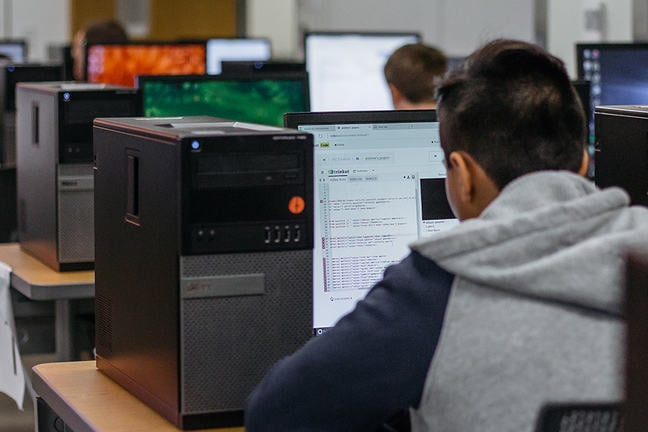## Want to keep learning?

This content is taken from the Raspberry Pi Foundation & National Centre for Computing Education's online course, How Computers Work: Demystifying Computation. Join the course to learn more.
3.8

## Raspberry Pi Foundation# Bits, Bytes and Nibbles

Before we proceed any further, let us have a quick look at the naming system that is commonly used when talking about binary numbers and data.

You already know that when counting in binary, you only use the digits 1 and 0. These are called the binary digits, which is normally abbreviated to bit.

So we often talk about bits of data, flipping bits, shifting bits etc. In each case, we are talking about manipulating a single binary digit.

Historically, when computers were first being designed, single characters of text were represented using eight bits.

So for instance the letter “a” was represented with the binary number 01100001, whereas the letter “Q” was represented by the number 01010001.

A collection of eight bits, therefore, came to be the smallest unit of storing data, and so deserved a name all of its own. A deliberate misspelling of bite was chosen, and so the name byte came into being, so it would not be confused with bit.

Keeping with the theme, a half byte (4 bits) was given the name nibble. This number of bits was fairly important in tiny computers called microprocessors.

For larger numbers of bits, we tend to use the standard scientific nomenclature for large multiples: kilo-, mega-, giga-, etc.

name symbol number of bits
bit b 1
nibble   4
byte B 8
kilobit kb 1000
kilobyte kB 8000
megabit Mb 1,000,000
megabyte MB 8,000,000

As a quick note, it is often useful to talk about a number of bits equal to a power of 2. In such cases, Kbit and Kibibyte (KiB) can be used to denote multiples of 1024 (a power of 2) instead of 1000. So 1Kbit is 1024 bits, and 1KiB is 1024 bytes.